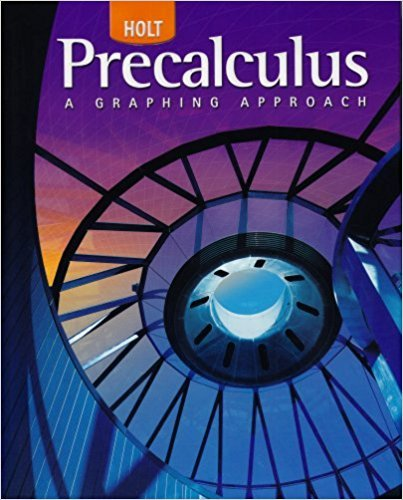×
×

# Solutions for Chapter 5.6: Solving Exponential and Logarithmic Equations## Full solutions for Precalculus | 1st Edition

ISBN: 9780030416477Solutions for Chapter 5.6: Solving Exponential and Logarithmic Equations

Solutions for Chapter 5.6
4 5 0 313 Reviews
12
0
##### ISBN: 9780030416477

This expansive textbook survival guide covers the following chapters and their solutions. Since 80 problems in chapter 5.6: Solving Exponential and Logarithmic Equations have been answered, more than 23864 students have viewed full step-by-step solutions from this chapter. Precalculus was written by and is associated to the ISBN: 9780030416477. This textbook survival guide was created for the textbook: Precalculus, edition: 1. Chapter 5.6: Solving Exponential and Logarithmic Equations includes 80 full step-by-step solutions.

Key Calculus Terms and definitions covered in this textbook
• Additive inverse of a real number

The opposite of b , or -b

• Continuous at x = a

lim x:a x a ƒ(x) = ƒ(a)

• Cotangent

The function y = cot x

• Coterminal angles

Two angles having the same initial side and the same terminal side

• Distance (in Cartesian space)

The distance d(P, Q) between and P(x, y, z) and Q(x, y, z) or d(P, Q) ((x ) - x 2)2 + (y1 - y2)2 + (z 1 - z 2)2

• Focal width of a parabola

The length of the chord through the focus and perpendicular to the axis.

• Histogram

A graph that visually represents the information in a frequency table using rectangular areas proportional to the frequencies.

• Hypotenuse

Side opposite the right angle in a right triangle.

• Inverse cosine function

The function y = cos-1 x

• Multiplication property of equality

If u = v and w = z, then uw = vz

• Ordered set

A set is ordered if it is possible to compare any two elements and say that one element is “less than” or “greater than” the other.

• Parametric curve

The graph of parametric equations.

• Probability simulation

A numerical simulation of a probability experiment in which assigned numbers appear with the same probabilities as the outcomes of the experiment.

• Recursively defined sequence

A sequence defined by giving the first term (or the first few terms) along with a procedure for finding the subsequent terms.

• Row operations

See Elementary row operations.

• Scalar

A real number.

• Singular matrix

A square matrix with zero determinant

• Standard form of a polar equation of a conic

r = ke 1 e cos ? or r = ke 1 e sin ? ,

• Terms of a sequence

The range elements of a sequence.

• Weights

See Weighted mean.

×